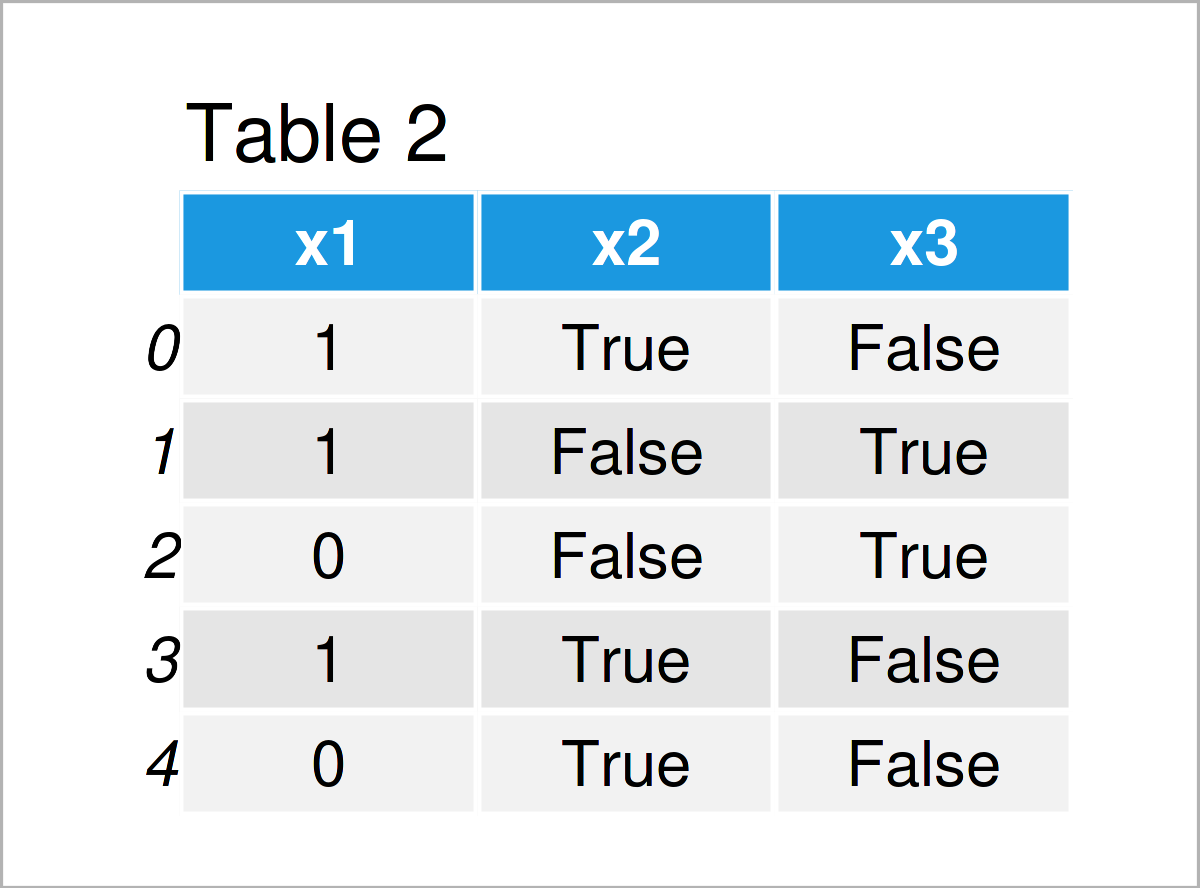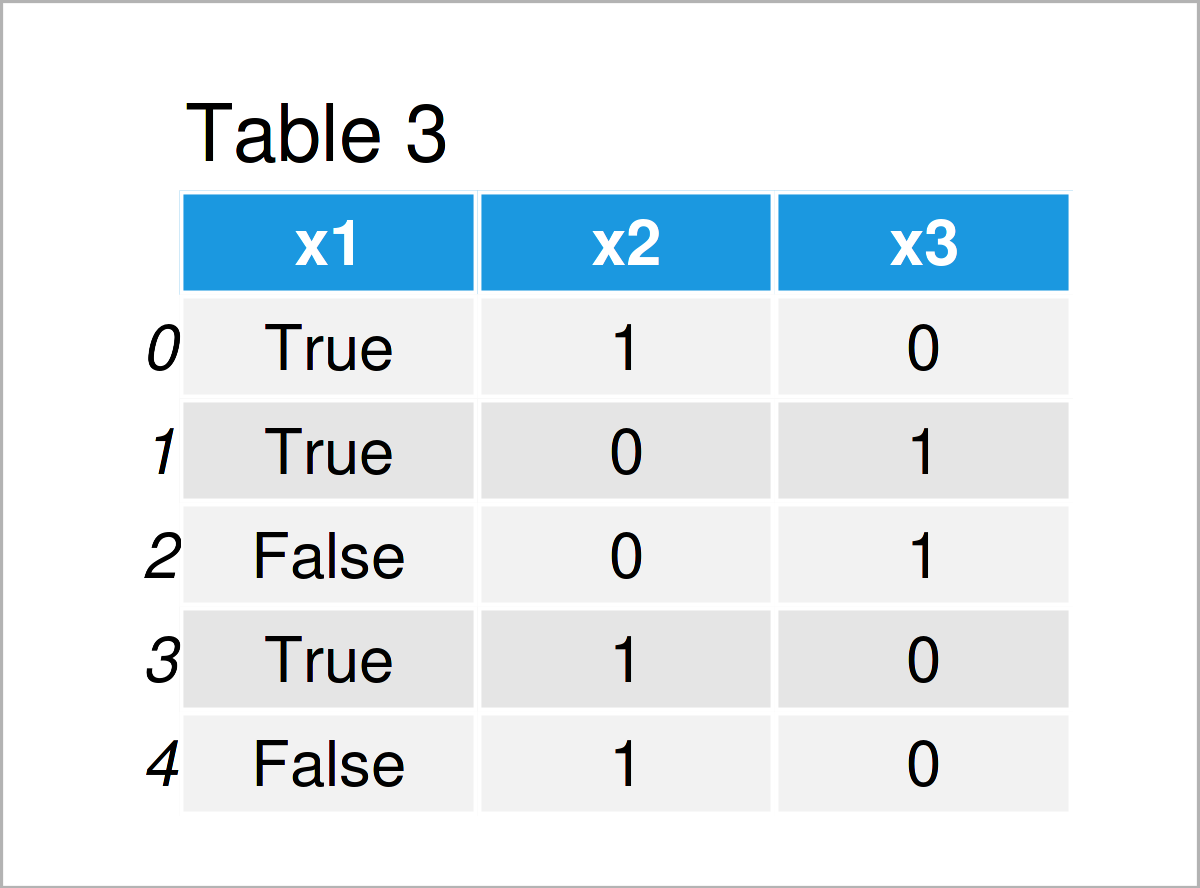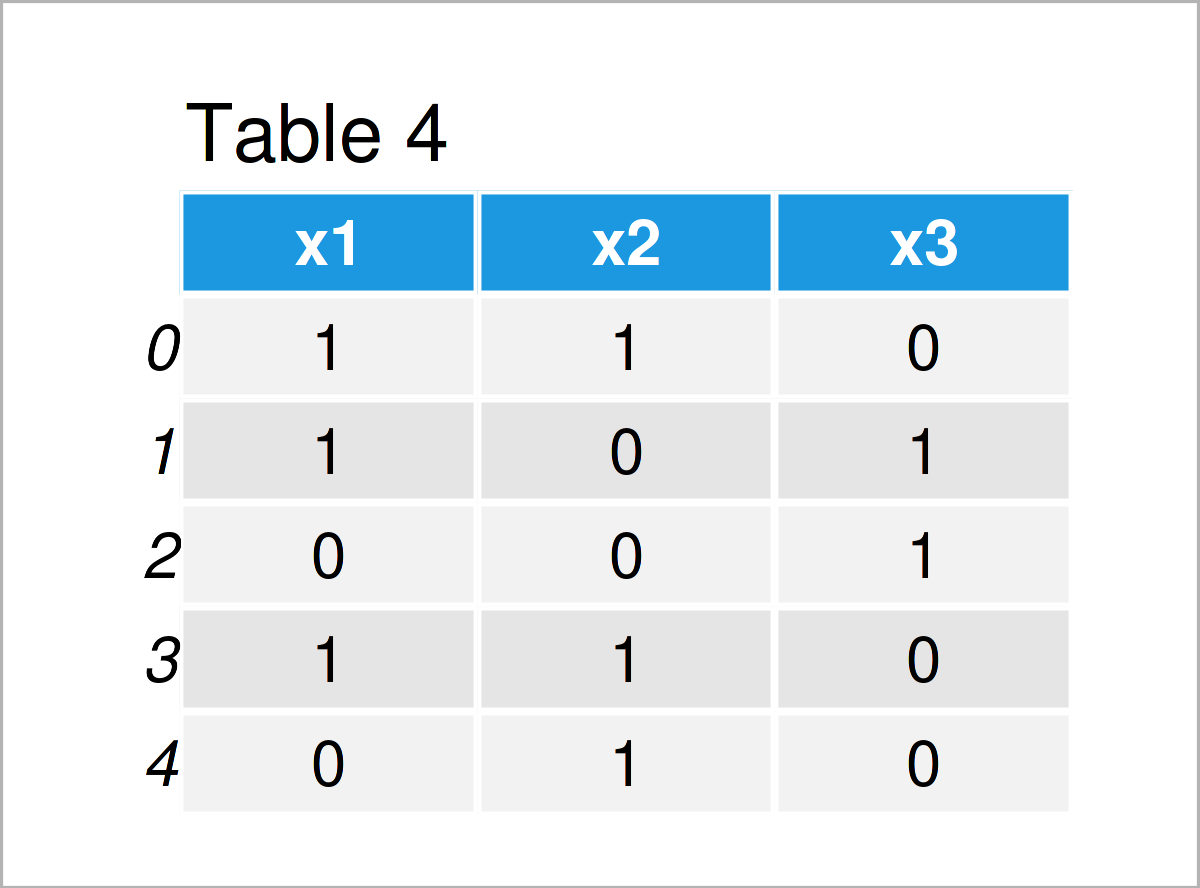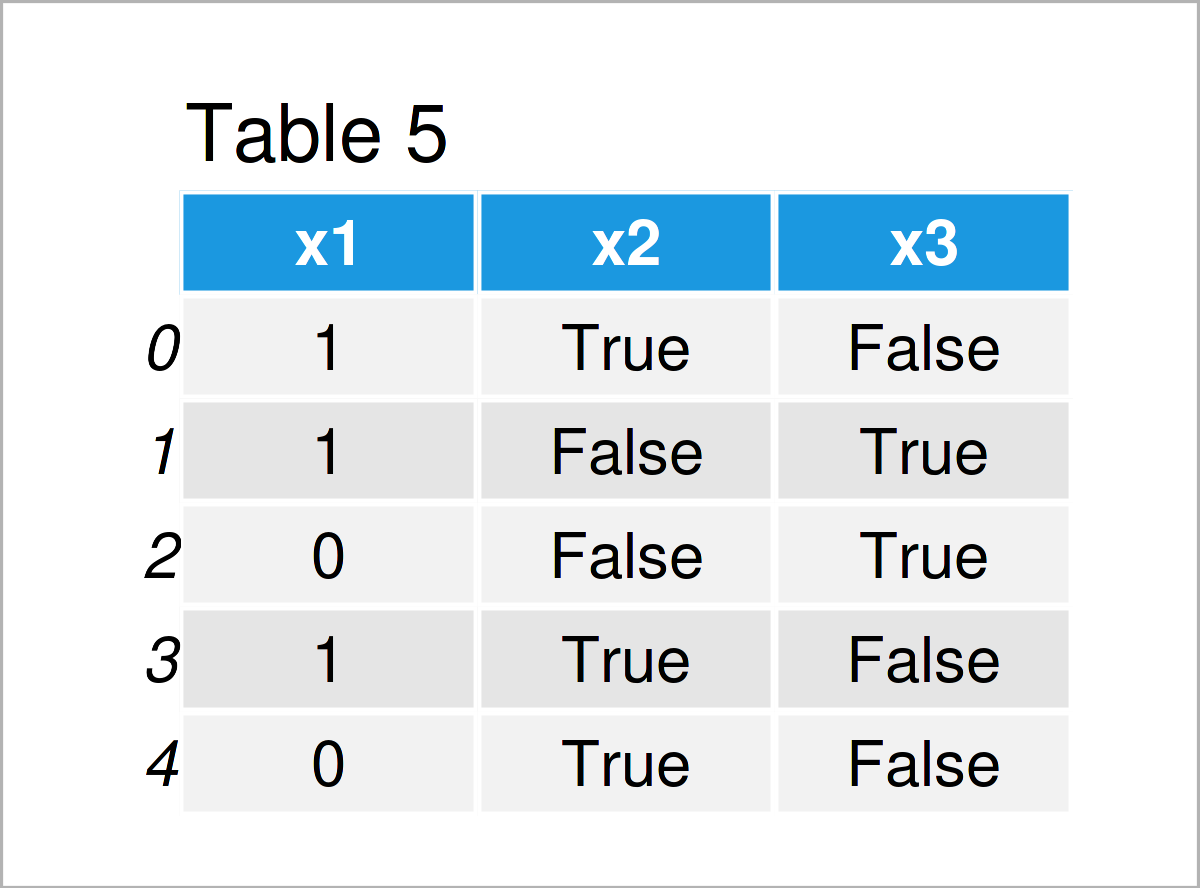# Convert True/False Boolean to 1/0 Dummy Integer in pandas DataFrame Column in Python (4 Examples)

On this page, I’ll illustrate how to convert a True/False boolean column to a 1/0 integer dummy in a pandas DataFrame in the Python programming language.

The tutorial will consist of these contents:

Let’s start right away.

## Example Data & Software Libraries

We first need to import the pandas library:

`import pandas as pd                                          # Load pandas library`

Furthermore, consider the following example data:

```data = pd.DataFrame({'x1':[True, True, False, True, False],  # Create pandas DataFrame
'x2':[True, False, False, True, True],
'x3':[False, True, True, False, False]})
print(data)                                                  # Print pandas DataFrame```As you can see based on Table 1, the example data is a pandas DataFrame containing five data points and three variables. As you can see, all elements in our example data are True or False logical indicators.

Let’s check the data types of the columns in our data set:

```print(data.dtypes)                                           # Check data types of columns
# x1    bool
# x2    bool
# x3    bool
# dtype: object```

As you can see, all the three columns in our pandas DataFrame are booleans.

## Example 1: Convert Single pandas DataFrame Column from Boolean to Integer

In Example 1, I’ll demonstrate how to change the data type of one specific column in a pandas DataFrame from boolean to integer.

To accomplish this, we can apply the astype function on one single column as shown below:

```data_new1 = data.copy()                                      # Create copy of DataFrame
data_new1['x1'] = data_new1['x1'].astype(int)                # Transform boolean to integer
print(data_new1)                                             # Print updated pandas DataFrame```As shown in Table 2, we have created a new pandas DataFrame called data_new1 by running the previous syntax. The first column of this DataFrame has been transformed to a 1/0 dummy with the integer class.

Let’s have a look at the dtypes of the columns in our new pandas DataFrame:

```print(data_new1.dtypes)                                      # Check data types of columns
# x1    int32
# x2     bool
# x3     bool
# dtype: object```

The first column has been converted to the integer class.

## Example 2: Convert Multiple pandas DataFrame Columns from Boolean to Integer

This example explains how to convert multiple columns in a pandas DataFrame from a True/False boolean to a 1/0 dummy indicator.

Once again, we can use the astype command for this task:

```data_new2 = data.copy()                                      # Create copy of DataFrame
data_new2 = data_new2.astype({'x2': int, 'x3': int})         # Transform multiple booleans to integer
print(data_new2)                                             # Print updated pandas DataFrame```As shown in Table 3, the previous Python programming code has created another pandas DataFrame where the values in the columns x2 and x3 have been switched from boolean to integer.

Let’s confirm that by printing the data types of our new columns:

```print(data_new2.dtypes)                                      # Check data types of columns
# x1     bool
# x2    int32
# x3    int32
# dtype: object```

Looks correct!

## Example 3: Convert All pandas DataFrame Columns from Boolean to Integer

The following Python code explains how to modify the data type of all columns in a pandas DataFrame.

If we want to convert all columns from boolean to integer, we can apply the astype function to the entire data set:

```data_new3 = data.copy()                                      # Create copy of DataFrame
data_new3 = data_new3.astype(int)                            # Transform all columns to integer
print(data_new3)                                             # Print updated pandas DataFrame```The output of the previous Python code is shown in Table 4 – A new pandas DataFrame containing only 1/0 dummy indicators.

Let’s have another look at the dtypes of our columns:

```print(data_new3.dtypes)                                      # Check data types of columns
# x1    int32
# x2    int32
# x3    int32
# dtype: object```

All variables in our data set are integers.

## Example 4: Convert pandas DataFrame Column from Boolean to Integer Using apply() Function

So far, we have only used the astype function to parse the variables in our pandas DataFrame from boolean to integer.

However, the Python programming language provides further functions to change the data types of pandas DataFrame columns.

In Example 4, I’ll show how to use the apply function to adjust the class of a specific DataFrame variable:

```data_new4 = data.copy()                                      # Create copy of DataFrame
data_new4['x1'] = data_new4['x1'].apply(int)                 # Transform boolean to integer
print(data_new4)                                             # Print updated pandas DataFrame```The output of the previous Python programming code is shown in Table 5: Another pandas DataFrame with one certain dummy column.

For completeness, let’s print the dtypes of our columns once again:

```print(data_new4.dtypes)                                      # Check data types of columns
# x1    int64
# x2     bool
# x3     bool
# dtype: object```

As you can see, we have converted only the first column to the integer data type.

## Video, Further Resources & Summary

I have recently released a video on the Statistics Globe YouTube channel, which illustrates the Python programming syntax of this tutorial. You can find the video below.

In addition, you may want to read the related articles that I have published on statisticsglobe.com. You can find a selection of articles on related topics such as groups, counting, and data conversion below:

In this Python tutorial you have learned how to convert a True/False boolean data type to a 1/0 integer dummy in a pandas DataFrame column.

Please note that we could have applied the same syntax to convert booleans to float columns. We only would have to specify float instead of int within the astype function.